###Author Topic: 16K RSA Keys in PGP  (Read 3633 times)

0 Members and 1 Guest are viewing this topic.

####Foxpup

• Hero Member
• Species: Cyborg Fox
••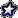•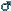• Posts: 1184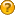##### 16K RSA Keys in PGP
« on: April 07, 2011, 12:18:44 am »
Given that 512 bit RSA keys have been cracked wide open ages ago, and that 1024 bit keys could possibly be cracked by the NSA (it's only 218.75 times harder, approximately), I find myself growing increasingly suspicious of the 4096 bit limit imposed by current PGP implementations. So I took it upon myself to make the following changes to my copy of GnuPG (version 1.4.11):

keygen.c  Line 1580  replace "max=4096" with "max=16384"
gpg.c     Line 2011  replace "secmem_init( 32768 )" with "secmem_init( 131072 )"

The first increases the arbitrary keysize limit to an even bigger arbitrary keysize limit. The second increases the amount of secure memory available (otherwise you'll run of memory generating the massive keys).

I generated a 16K/16K RSA v4 test key without any problems other than accumulating the several kilobytes of entropy required (I really need to get a hardware entropy gatherer...) The test key works fine, even on unmodified PGP implementations (as it should, since the OpenPGP standard doesn't say anything about keysize limits (except for DSA keys, but that's another story)). The key uses AES256 cipher and SHA256 digest algorithms (anything less would defeat the whole purpose of having a gigantic RSA key, if Bruce Schneier is to believed (and he isn't*)). I'd post the key, but it's freakin' huge - nearly 400 lines long.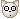Anyway, as with any "minor" change to cryptographic software that appears to work correctly, deadly unforeseen consequences are likely to explode in my face (although I don't think this is anything like the Debian OpenSSL fiasco...) I'm mostly worried about prime generation - does GnuPG generate primes of this size correctly? It probably does, but it would be dangerous to simply assume that. How can I be sure? Also, do I look like a crazy person just for suggesting this?

*Bruce Schneier says that gigantic asymmetric keys are useless because it's still just as easy to break the underlying symmetric key (or maybe I misinterpreted?). This is clearly bogus because breaking the symmetric key is only good for that one message, but breaking the asymmetric key gives you all messages that ever have and ever will be encrypted using that key. So it's perfectly reasonable (as far as I can tell) to use an asymmetric cipher that's vastly more secure than the symmetric cipher.
Hmm... They have the Internet on computers now. - Homer Simpson

Art doesn't work without pain. Art exists for compensating pain. - Till Lindemann

There's a fine line between sayings that make sense. - Too Much Coffee Man

####redyoshi49q##### Re: 16K RSA Keys in PGP
« Reply #1 on: April 11, 2011, 07:38:17 pm »
You know a lot more about this topic than I do, and I'm not an authority on this subject by any means.  Having said that, I found these two descriptions of GPG prime generation.

From what I can tell, GPG doesn't *prove* that the numbers it generates are prime; it finds numbers that are "probably" prime.  If the consideration of larger primes leads to the greater likelihood of a false positive on the primality tests, then it seems like increasing the iterations of the primality tests would maintain the high chance of actually generating a prime.  Additionally, it may be prudent to test more low primes as well (maybe primes up to 50k/500k?).  Aside from that, it doesn't seem that the prime generating algorithm would have issues with generating arbitrarily large primes.

...I'm confused as to what exactly you're worried about as well.  Wouldn't an imperfect prime generator simply generate composite numbers on occasion, and thus occasionally produce keypairs that would distort almost all of the data they process?  It's far from desirable, but the issue is immediately noticeable upon an attempted decryption, and for even an adequate prime generator, all you'd have to do is generate another keypair (or if you're *really* unlucky, two).  From a usability standpoint, it's horrendous, but it doesn't seem to affect things from a security standpoint when it comes to keys that are manually verified as working.  I feel like I'm missing something important...

If this does work without any practical or theoretical hitches whatsoever, it makes me question the need of the upper limit in the first place.  It might be better to have an upper limit calculated from a cursory glance at computer resources, then to initialize the secure memory calculated from the generated key size...
"Perfect normality is impossible.  Be unique!"
-- redyoshi49q^ (click) Puzzle game!

####Foxpup

• Hero Member
• Species: Cyborg Fox
•••• Posts: 1184##### Re: 16K RSA Keys in PGP
« Reply #2 on: April 11, 2011, 09:18:24 pm »
You know a lot more about this topic than I do, and I'm not an authority on this subject by any means.  Having said that, I found these two descriptions of GPG prime generation.

From what I can tell, GPG doesn't *prove* that the numbers it generates are prime; it finds numbers that are "probably" prime.  If the consideration of larger primes leads to the greater likelihood of a false positive on the primality tests, then it seems like increasing the iterations of the primality tests would maintain the high chance of actually generating a prime.  Additionally, it may be prudent to test more low primes as well (maybe primes up to 50k/500k?).  Aside from that, it doesn't seem that the prime generating algorithm would have issues with generating arbitrarily large primes.
Yes, I am aware that PGP doesn't prove that its primes really are prime. For a 1024 bit key (using 512 bit primes), the chances that a "prime" is actually composite are 10-44, which is very slim indeed. The problem is, are larger primes much more likely to be composite, and if so, are these pseudoprimes worse than smaller primes in terms of ease of factorisation?

...Though I don't know what you mean by primes up to 50k/500k. If you're talking about number of bits, those are ludicrously large primes! If you're talking about actual integer values, then they're ludicrously small - a 4k RSA key uses primes with 612 decimal digits!

...I'm confused as to what exactly you're worried about as well.  Wouldn't an imperfect prime generator simply generate composite numbers on occasion, and thus occasionally produce keypairs that would distort almost all of the data they process?  It's far from desirable, but the issue is immediately noticeable upon an attempted decryption, and for even an adequate prime generator, all you'd have to do is generate another keypair (or if you're *really* unlucky, two).  From a usability standpoint, it's horrendous, but it doesn't seem to affect things from a security standpoint when it comes to keys that are manually verified as working.  I feel like I'm missing something important...
You are. Composite numbers will seem to work fine, in that you can create a key-pair with them and use it to encrypt and decrypt. But there's a problem. To see why composite numbers are bad, here's how the an RSA key is generated, in three easy steps:
Step 1: Create two prime numbers, p and q, and multiply them to create the modulus, n = pq.
Step 2: Create a third number, e, that is relatively prime to (is not divisible by) the product (p-1)(q-1). This is the public exponent.
Step 3: Calculate an integer d, such that the quotient (ed-1)/((p-1)(q-1)) is an integer. This is the private exponent.

The public key consists of the modulus and the public exponent (n,e), and the private key consists of the modulus and the private exponent (n,d). For encryption, the ciphertext, C, is created from the plaintext, M, using the public key (n,e), thusly:
C=Me mod n
For decryption, you just repeat using the private key:
M=Cd mod n

The plaintext, in most applications (including PGP), is a randomly generated key to a symmetric cipher (such as AES), which is used to encrypt the actual message, because symmetric ciphers are so much faster for the same level of security.

Now for the big problem. Can an attacker calculate the private key (n,d) from the public key (n,e)? (If he can, the key is obviously broken) All he has to do is perform step 3 above, which requires him to know the values of p and q (which requires that factorise n, which is widely assumed to be an NP-hard problem). At least, he does if p and q are primes. It actually works with any values of p and q such that pq = n. As long as p and q are prime, they are only values that work, but if p and q are composite, their factors will work just as well. Or, to put it more simply, factorising a number which is the product of two primes is hard, but factorising a number that is the product of two composites is easy (or at least easier, depending on how big the prime factors of the composites are).

TL;DR: Composites are bad, in that they make it much easier for someone to break your private key. However, they still encrypt and decrypt normally, so you don't know they're bad until it's too late.

If this does work without any practical or theoretical hitches whatsoever, it makes me question the need of the upper limit in the first place.  It might be better to have an upper limit calculated from a cursory glance at computer resources, then to initialize the secure memory calculated from the generated key size...
Theoretical hitches first - an asymmetric key is only as strong as the underlying symmetric cipher and digest algorithms (but see the last paragraph of my first post). Back when 128 bit symmetric ciphers where used, anything more than a 4096 bit asymmetric key was overkill. But now everyone is using (or ought to be using) 256 bit asymmetric ciphers, which is ridiculous - it is much, much easier to break a 4096 bit asymmetric key than the 256 bit symmetric key, so the 256 bit key doesn't buy you any extra security over a 128 bit key, unless you use a huge asymmetric key.
Practical hitches - huge keys require a correspondingly huge amount of entropy to generate. A 16k key requires more entropy than most computers can generate in a reasonable period of time (generating the test key took 10 minutes on a 3.2GHz Phenom II X6). But that's only to generate the keys - merely using huge keys is slower than smaller keys, but not excessively so. This may be an issue on mobile devices with pathetically slow CPUs, but otherwise it's not a problem. Hmm...
Hmm... They have the Internet on computers now. - Homer Simpson

Art doesn't work without pain. Art exists for compensating pain. - Till Lindemann

There's a fine line between sayings that make sense. - Too Much Coffee Man

####redyoshi49q##### Re: 16K RSA Keys in PGP
« Reply #3 on: April 11, 2011, 11:45:30 pm »
...Though I don't know what you mean by primes up to 50k/500k. If you're talking about number of bits, those are ludicrously large primes! If you're talking about actual integer values, then they're ludicrously small - a 4k RSA key uses primes with 612 decimal digits!

I did mean integer values.  The second page I linked said that the GPG first checks a possible prime against all primes less than 5k before resorting to more theoretical tests, and I guessed that 50k/500k might be adequate starting places for this practice on larger possible prime numbers.  Since there are other tests, the stopping point for testing individual primes can be small.

You are. Composite numbers will seem to work fine, in that you can create a key-pair with them and use it to encrypt and decrypt. But there's a problem. To see why composite numbers are bad, here's how the an RSA key is generated, in three easy steps:
Step 1: Create two prime numbers, p and q, and multiply them to create the modulus, n = pq.
Step 2: Create a third number, e, that is relatively prime to (is not divisible by) the product (p-1)(q-1). This is the public exponent.
Step 3: Calculate an integer d, such that the quotient (ed-1)/((p-1)(q-1)) is an integer. This is the private exponent.

The public key consists of the modulus and the public exponent (n,e), and the private key consists of the modulus and the private exponent (n,d). For encryption, the ciphertext, C, is created from the plaintext, M, using the public key (n,e), thusly:
C=Me mod n
For decryption, you just repeat using the private key:
M=Cd mod n

[. . .]

Now for the big problem. Can an attacker calculate the private key (n,d) from the public key (n,e)? (If he can, the key is obviously broken) All he has to do is perform step 3 above, which requires him to know the values of p and q (which requires that factorise n, which is widely assumed to be an NP-hard problem). At least, he does if p and q are primes. It actually works with any values of p and q such that pq = n. As long as p and q are prime, they are only values that work, but if p and q are composite, their factors will work just as well. Or, to put it more simply, factorising a number which is the product of two primes is hard, but factorising a number that is the product of two composites is easy (or at least easier, depending on how big the prime factors of the composites are).

TL;DR: Composites are bad, in that they make it much easier for someone to break your private key. However, they still encrypt and decrypt normally, so you don't know they're bad until it's too late.

I did a few tests in Python.  The first was a "make sure I know what I'm doing" test (you can skip this if you want), and the second two are "see what happens when p and q are not both prime" tests.

Code: [Select]
`Case 1 (simple case w/ 2 primes):>>> p = 3>>> q = 7>>> n = p*q>>> n21>>> phi = (p-1)*(q-1)>>> phi12>>> e = 5>>> (5 * 5) % 121>>> d = 5>>> def c(m, e=5, n=21):...  return ( (m**e) % n)... >>> def m(c, d=5, n=21):...  return ( (c**d) % n)... >>> count = 0>>> for i in range(1, 21):...  i, c(i), m(c(i))...  if (i == m(c(i)) ):...   count = count + 1... (1, 1, 1)(2, 11, 2)(3, 12, 3)(4, 16, 4)(5, 17, 5)(6, 6, 6)(7, 7, 7)(8, 8, 8)(9, 18, 9)(10, 19, 10)(11, 2, 11)(12, 3, 12)(13, 13, 13)(14, 14, 14)(15, 15, 15)(16, 4, 16)(17, 5, 17)(18, 9, 18)(19, 10, 19)(20, 20, 20)>>> count20Case 2 (simple case w/ a composite number):>>> p = 5>>> q = 14>>> n = p*q>>> n70>>> phi = (p-1)*(q-1)>>> phi52>>> e = 11>>> (19 * 11) % 521>>> d = 19>>> def c(m, e=11, n=70):...  return ( (m**e) % n)... >>> def m(c, d=19, n=70):...  return ( (c**d) % n)... >>> count = 0>>> for i in range(1, 70):...  i, c(i), m(c(i))...  if (i == m(c(i)) ):...   count = count + 1... (1, 1, 1)(2, 18, 32L)(3, 47, 33L)(4, 44, 44L)(5, 45, 45L)(6, 6, 6)(7, 63, 7L)(8, 22, 8L)(9, 39, 39L)(10, 40, 40L)(11, 51, 51L)(12, 38, 52L)(13, 27, 13L)(14, 14, 14L)(15, 15, 15L)(16, 46, 46L)(17, 33, 47L)(18, 2, 58)(19, 59, 59L)(20, 20, 20L)(21, 21, 21L)(22, 8, 22)(23, 67, 53L)(24, 54, 54L)(25, 65, 65L)(26, 66, 66L)(27, 13, 27L)(28, 42, 28L)(29, 29, 29L)(30, 60, 60L)(31, 61, 61L)(32, 58, 2L)(33, 17, 3L)(34, 34, 34L)(35, 35, 35L)(36, 36, 36L)(37, 53, 67L)(38, 12, 68L)(39, 9, 9)(40, 10, 10L)(41, 41, 41L)(42, 28, 42L)(43, 57, 43L)(44, 4, 4)(45, 5, 5)(46, 16, 16L)(47, 3, 17)(48, 62, 48L)(49, 49, 49L)(50, 50, 50L)(51, 11, 11L)(52, 68, 12L)(53, 37L, 23L)(54, 24L, 24L)(55, 55L, 55L)(56, 56L, 56L)(57, 43L, 57L)(58, 32L, 18L)(59, 19L, 19L)(60, 30L, 30L)(61, 31L, 31L)(62, 48L, 62L)(63, 7L, 63L)(64, 64L, 64L)(65, 25L, 25L)(66, 26L, 26L)(67, 23L, 37L)(68, 52L, 38L)(69, 69L, 69L)>>> count29Case 3 (slightly more complex case w/ 1 composite):>>> p = 31>>> q = 17 * 19>>> q323>>> n = p*q>>> n10013>>> phi = (p-1)*(q-1)>>> phi9660>>> e = 257>>> for i in range(1, 9660):...  if (i * e) % phi == 1:...   i... 6653>>> d = 6653>>> def c(m, e=257, n=10013):...  return ( (m**e) % n)... >>> def m(c, d=6653, n=10013):...  return ( (c**d) % n)... >>> for i in range(1, 100):...  i, c(i), m(c(i))... (1, 1, 1)(2, 4592L, 7070L)(3, 8299L, 4126L)(4, 9099L, 4L)(5, 9015L, 3539L)(6, 9543L, 2951L)(7, 4172L, 1774L)(8, 8372L, 8254L)(9, 3987L, 1776L)(10, 3138L, 8256L)(11, 7474L, 7079L)(12, 4568L, 6491L)(13, 3396L, 13L)(14, 2955L, 5904L)(15, 8362L, 2960L)(16, 4317L, 16L)(17, 8670L, 17L)(18, 4540L, 18L)(19, 3895L, 7087L)(20, 989L, 4143L)(21, 8487L, 21L)(22, 6057L, 3556L)(23, 5888L, 2968L)(24, 9034L, 1791L)(25, 4717L, 8271L)(26, 4191L, 1793L)(27, 5161L, 8273L)(28, 1745L, 7096L)(29, 7033L, 6508L)(30, 8462L, 30L)(31, 8463L, 5921L)(32, 7937L, 2977L)(33, 6204L, 33L)(34, 952L, 34L)(35, 1752L, 35L)(36, 614L, 7104L)(37, 7466L, 4160L)(38, 2622L, 38L)(39, 6822L, 3573L)(40, 5599L, 2985L)(41, 3169L, 1808L)(42, 1708L, 8288L)(43, 7761L, 1810L)(44, 7643L, 8290L)(45, 6148L, 7113L)(46, 2596L, 6525L)(47, 4348L, 47L)(48, 269L, 5938L)(49, 2990L, 2994L)(50, 2345L, 50L)(51, 8925L, 51L)(52, 86L, 52L)(53, 8723L, 7121L)(54, 8554L, 4177L)(55, 633L, 55L)(56, 2640L, 3590L)(57, 2641L, 3002L)(58, 3611L, 1825L)(59, 3357L, 8305L)(60, 7064L, 1827L)(61, 2696L, 8307L)(62, 1643L, 7130L)(63, 2171L, 6542L)(64, 9397L, 64L)(65, 5199L, 5955L)(66, 1783L, 3011L)(67, 2226L, 67L)(68, 5916L, 68L)(69, 1072L, 69L)(70, 4745L, 7138L)(71, 7211L, 4194L)(72, 5835L, 72L)(73, 4374L, 3607L)(74, 9373L, 3019L)(75, 5566L, 1842L)(76, 4598L, 8322L)(77, 1046L, 1844L)(78, 5960L, 8324L)(79, 300L, 7147L)(80, 7237L, 6559L)(81, 5538L, 81L)(82, 3159L, 5972L)(83, 3279L, 3028L)(84, 2957L, 84L)(85, 8585L, 85L)(86, 2245L, 86L)(87, 1090L, 7155L)(88, 1091L, 4211L)(89, 1534L, 89L)(90, 4969L, 3624L)(91, 9730L, 3036L)(92, 5362L, 1859L)(93, 3255L, 8339L)(94, 94L, 1861L)(95, 7847L, 8341L)(96, 3649L, 7164L)(97, 3395L, 6576L)(98, 2257L, 98L)(99, 150L, 5989L)>>> count = 0>>> for i in range(1, 10013):...  if (i == m(c(i)) ):...   count = count + 1... >>> count2944`
The triple value lists in each test show message values, the corresponding sent (encrypted) values, and the corresponding received (decrypted) values for many or all of the possible message values for that system.  The count variables count the number of received values that equal their corresponding message values (in a working system, this should equal n-1).

As the last two tests show, the system pretty much falls apart* if p and q are not both selected to be prime (unless a list of values of M that won't be distorted by the cryptosystem was compiled for each keypair so that only such M values would be sent by that keypair, but that seems a tad ridiculous and possibly insecure).  Since the system doesn't work anyway, the relatively increased ease of factoring n is irrelevant.  Also, since m(c(M)) != M for a substantial proportion of possible M, a generated false prime should lead to a failed encrypt/decrypt test, which should in turn prompt the accordingly informed user to generate another keypair.  Again, although a pain from the user's perspective, there doesn't seem to be a security issue with accidentally generating an occasional composite number in practice, assuming said composites are sufficiently infrequent to eventually generate a keypair..

*I obviously cannot test every such prime p and nonprime q, and I don't yet have the mathemagical (sp) knowledge to prove this for every or almost every prime p and nonprime q.  Thus, I could still see a slight possibility of this not happening for some really large composite number(s).

(*edit*) I massively goofed up the first case, and I've updated it to use the values I originally intended (the ones I used on pencil and paper).  The goofed up first case is below:

Code: [Select]
`>>> p = 3>>> q = 14>>> n = p*q>>> n42>>> phi = (p-1)*(q-1)>>> phi26>>> e = 5>>> (5 * 5) % 241>>> d = 5>>> def c(m, e=5, n=42):...  return ( (m**e) % n)... >>> def m(c, d=5, n=42):...  return ( (c**d) % n)... >>> count = 0>>> for i in range(1, 42):...  i, c(i), m(c(i))...  if (i == m(c(i)) ):...   count = count + 1... (1, 1, 1)(2, 32, 2)(3, 33, 3)(4, 16, 4)(5, 17, 5)(6, 6, 6)(7, 7, 7)(8, 8, 8)(9, 39, 9)(10, 40, 10)(11, 23, 11)(12, 24, 12)(13, 13, 13)(14, 14, 14)(15, 15, 15)(16, 4, 16)(17, 5, 17)(18, 30, 18)(19, 31, 19)(20, 20, 20)(21, 21, 21)(22, 22, 22)(23, 11, 23)(24, 12, 24)(25, 37, 25)(26, 38, 26)(27, 27, 27)(28, 28, 28)(29, 29, 29)(30, 18, 30)(31, 19, 31)(32, 2, 32)(33, 3, 33)(34, 34, 34)(35, 35, 35)(36, 36, 36)(37, 25, 37)(38, 26, 38)(39, 9, 39)(40, 10, 40)(41, 41, 41)>>> count41`
Strangely enough, it somehow *did* work out to be a functioning crypto system, in spite of several things that went wrong with the algorithm (among them, the compositeness of q and the accident in the choice of d (in other news, 24 now equals 26) ).  So in effect, this lends some more credibility to the possibility of GPG working in spite of using composites for p and q (though it doesn't prove this, as the real modular inverse of 5 here was 21).

(*heads to bed, since he obviously needs sleep*)

(*edit again*) I messed up the third case as well.  I took the modular inverse of e modulo n instead of modulo phi, and therefore got 7013 for d instead of 6653.  That example has also been fixed.
« Last Edit: April 12, 2011, 10:20:04 am by redyoshi49q »
"Perfect normality is impossible.  Be unique!"
-- redyoshi49q^ (click) Puzzle game!

####Foxpup

• Hero Member
• Species: Cyborg Fox
•••• Posts: 1184##### Re: 16K RSA Keys in PGP
« Reply #4 on: April 13, 2011, 12:58:44 am »
...Though I don't know what you mean by primes up to 50k/500k. If you're talking about number of bits, those are ludicrously large primes! If you're talking about actual integer values, then they're ludicrously small - a 4k RSA key uses primes with 612 decimal digits!

I did mean integer values.  The second page I linked said that the GPG first checks a possible prime against all primes less than 5k before resorting to more theoretical tests, and I guessed that 50k/500k might be adequate starting places for this practice on larger possible prime numbers.  Since there are other tests, the stopping point for testing individual primes can be small.
No it doesn't. It checks to see if the candidate prime is divisible by all primes less than 5000. Obviously, if a number is divisible by any other number, it's not prime; and if a number if divisible by a composite number, it's also divisible by the prime factors of that number, so we only need to check prime numbers as divisors.

As the last two tests show, the system pretty much falls apart* if p and q are not both selected to be prime (unless a list of values of M that won't be distorted by the cryptosystem was compiled for each keypair so that only such M values would be sent by that keypair, but that seems a tad ridiculous and possibly insecure).  Since the system doesn't work anyway, the relatively increased ease of factoring n is irrelevant.  Also, since m(c(M)) != M for a substantial proportion of possible M, a generated false prime should lead to a failed encrypt/decrypt test, which should in turn prompt the accordingly informed user to generate another keypair.  Again, although a pain from the user's perspective, there doesn't seem to be a security issue with accidentally generating an occasional composite number in practice, assuming said composites are sufficiently infrequent to eventually generate a keypair..

*I obviously cannot test every such prime p and nonprime q, and I don't yet have the mathemagical (sp) knowledge to prove this for every or almost every prime p and nonprime q.  Thus, I could still see a slight possibility of this not happening for some really large composite number(s).
But we're not talking about just any composites, we're talking about composites that pass various primality tests, aka pseudoprimes. Possibly, these pseudoprimes are more likely to work normally. I don't know. I'm not a mathemagician either.

(*edit*) I massively goofed up the first case, and I've updated it to use the values I originally intended (the ones I used on pencil and paper).  The goofed up first case is below:

....

Strangely enough, it somehow *did* work out to be a functioning crypto system, in spite of several things that went wrong with the algorithm (among them, the compositeness of q and the accident in the choice of d (in other news, 24 now equals 26) ).  So in effect, this lends some more credibility to the possibility of GPG working in spite of using composites for p and q (though it doesn't prove this, as the real modular inverse of 5 here was 21).
You're right, it does work, except for d being the same as e (which makes it a symmetric cipher, which is totally not we want).

(*heads to bed, since he obviously needs sleep*)
That would be a good idea.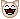Anyway, after going through the primegen.c I don't see anything that would suggest any problems with generating large primes (other than memory usage and CPU time). Which leaves us with the mystery of why the limit is there at all.
Hmm... They have the Internet on computers now. - Homer Simpson

Art doesn't work without pain. Art exists for compensating pain. - Till Lindemann

There's a fine line between sayings that make sense. - Too Much Coffee Man

####redyoshi49q##### Re: 16K RSA Keys in PGP
« Reply #5 on: April 13, 2011, 03:26:32 am »
I did mean integer values.  The second page I linked said that the GPG first checks a possible prime against all primes less than 5k before resorting to more theoretical tests, and I guessed that 50k/500k might be adequate starting places for this practice on larger possible prime numbers.  Since there are other tests, the stopping point for testing individual primes can be small.
No it doesn't. It checks to see if the candidate prime is divisible by all primes less than 5000. Obviously, if a number is divisible by any other number, it's not prime; and if a number if divisible by a composite number, it's also divisible by the prime factors of that number, so we only need to check prime numbers as divisors.

I did mean "divisibility checks" when I said "checking against".  Sorry that I wasn't more clear.

But we're not talking about just any composites, we're talking about composites that pass various primality tests, aka pseudoprimes. Possibly, these pseudoprimes are more likely to work normally. I don't know. I'm not a mathemagician either.

Code: [Select]
`Case 4 (w/ a composite, but a correctly computed totient instead of an assumed (p-1)*(q-1) ):>>> p = 31>>> q = 17 * 19>>> q323>>> n = p*q>>> n10013>>> phi = (p-1)*(17-1)*(19-1)>>> phi8640>>> e = 257>>> for i in range(1, 9660):...  if (i * e) % phi == 1:...   i... 4673>>> d = 4673>>> def c(m, e=257, n=10013):...  return ( (m**e) % n)... >>> def m(c, d=4673, n=10013):...  return ( (c**d) % n)... >>> for i in range(1, 100):...  i, c(i), m(c(i))... (1, 1, 1)(2, 4592L, 2L)(3, 8299L, 3L)(4, 9099L, 4L)(5, 9015L, 5L)(6, 9543L, 6L)(7, 4172L, 7L)(8, 8372L, 8L)(9, 3987L, 9L)(10, 3138L, 10L)(11, 7474L, 11L)(12, 4568L, 12L)(13, 3396L, 13L)(14, 2955L, 14L)(15, 8362L, 15L)(16, 4317L, 16L)(17, 8670L, 17L)(18, 4540L, 18L)(19, 3895L, 19L)(20, 989L, 20L)(21, 8487L, 21L)(22, 6057L, 22L)(23, 5888L, 23L)(24, 9034L, 24L)(25, 4717L, 25L)(26, 4191L, 26L)(27, 5161L, 27L)(28, 1745L, 28L)(29, 7033L, 29L)(30, 8462L, 30L)(31, 8463L, 31L)(32, 7937L, 32L)(33, 6204L, 33L)(34, 952L, 34L)(35, 1752L, 35L)(36, 614L, 36L)(37, 7466L, 37L)(38, 2622L, 38L)(39, 6822L, 39L)(40, 5599L, 40L)(41, 3169L, 41L)(42, 1708L, 42L)(43, 7761L, 43L)(44, 7643L, 44L)(45, 6148L, 45L)(46, 2596L, 46L)(47, 4348L, 47L)(48, 269L, 48L)(49, 2990L, 49L)(50, 2345L, 50L)(51, 8925L, 51L)(52, 86L, 52L)(53, 8723L, 53L)(54, 8554L, 54L)(55, 633L, 55L)(56, 2640L, 56L)(57, 2641L, 57L)(58, 3611L, 58L)(59, 3357L, 59L)(60, 7064L, 60L)(61, 2696L, 61L)(62, 1643L, 62L)(63, 2171L, 63L)(64, 9397L, 64L)(65, 5199L, 65L)(66, 1783L, 66L)(67, 2226L, 67L)(68, 5916L, 68L)(69, 1072L, 69L)(70, 4745L, 70L)(71, 7211L, 71L)(72, 5835L, 72L)(73, 4374L, 73L)(74, 9373L, 74L)(75, 5566L, 75L)(76, 4598L, 76L)(77, 1046L, 77L)(78, 5960L, 78L)(79, 300L, 79L)(80, 7237L, 80L)(81, 5538L, 81L)(82, 3159L, 82L)(83, 3279L, 83L)(84, 2957L, 84L)(85, 8585L, 85L)(86, 2245L, 86L)(87, 1090L, 87L)(88, 1091L, 88L)(89, 1534L, 89L)(90, 4969L, 90L)(91, 9730L, 91L)(92, 5362L, 92L)(93, 3255L, 93L)(94, 94L, 94L)(95, 7847L, 95L)(96, 3649L, 96L)(97, 3395L, 97L)(98, 2257L, 98L)(99, 150L, 99L)>>> count = 0>>> for i in range(1, 10013):...  if (i == m(c(i)) ):...   count = count + 1... >>> count10012`
So in summary, composite numbers can be made to work in RSA, but it requires a *correctly* calculated totient value for p*q to make this happen.  If p and q are assumed to be prime when they're not, then the calculated totient value will be messed up accordingly.  For instance, if q actually has factors r and s (p, r, and s are prime), then phi = (p-1)*(r-1)*(s-1) = (p-1)*(rs-r-s+1) = (p-1)*(q-1 -r-s+2) = (p-1)*(q-1) - (p-1)*(r+s-2).  The variables d and e are selected with the wrong phi value; therefore, d*e % ( (p-1)*(q-1) ) = 1.   But if d*e % ( (p-1)*(q-1) - (p-1)*(r+s-2) ) != 1, then the RSA algorithm won't work for all inputs (see "Consise proof using Euler's Theorem" on the RSA Wikipedia page).  The chances of some product d*e satisfying both the real and fake totient modulos by mere chance seems ridiculously unlikely by intuition.

I did a little bit of algebra on that as well, but it's only suggestive, not conclusive...

Code: [Select]
`a=q-1, c = p-1, b = r+s-2, q = r*sd*e = 1 + k0*a*cd*e = 1 + k1*(a*c - b*c)k0*a*c = k1*(a*c - b*c)k0 = (k1*(a-b) ) / ak0 = k1 - k1*b/ak0 = k1 - k1*( (r+s-2)/(r*s-1) )`
We know that if d and e are generated by the first modulus, then k0 exists and is a specific and finite but as-of-yet uncalculated integer.  If the second modulus is also true, then there must exist some integer k1 that satisfies the final equation.  However, the ( (r+s-2)/(r*s-1) ) term is about equal to the inverse of the lesser of r and s.  Therefore, k1 would probably have to be approximately equal to some unknown but positive multiple of the lesser of r and s (to make the second fraction an integer), yet small enough such that k1 wasn't too much greater than the finite k0 (to make the two sides of the equation have equal value).  Given the limits on d and e, k0 could only be as great as (p-1)*(q-1) in the worst case; if k1 needs to be too much larger to make the right side an integer, then the right side is too large to equal k0.  Again, it's far from a proof, but it's better than nothing.

Ironically, the totient thing also explains how the messed up example worked.  In that example, the "real" phi value was 12 ( (3-1)*(7-1)*(2-1)=12 ), and (5*5)%12 = 1.

...I think I'm starting to go a little too overboard on this topic...
"Perfect normality is impossible.  Be unique!"
-- redyoshi49q^ (click) Puzzle game!

####Foxpup

• Hero Member
• Species: Cyborg Fox
•••• Posts: 1184##### Re: 16K RSA Keys in PGP
« Reply #6 on: April 13, 2011, 10:23:49 pm »
*re-reads Wikipedia article on Euler's totient function, then bashes head against desk repeatedly*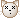Yes, that makes a lot more sense. So there's nothing to worry about with regards to choosing a composite instead of a prime (assuming the totient function is correctly implemented - from what I now understand, it would be easy to come up a bogus key generator that deliberately uses composites instead of primes, and the resulting key will work normally with "real" encryption software but be ludicrously easy to factor, making the NSA's job much easier, with no way to know whether a given key is bogus... *puts on tinfoil hat*). So it would appear that 16k keys are good, and that the 4k limit is there for... no reason at all? That can't be right...
Hmm... They have the Internet on computers now. - Homer Simpson

Art doesn't work without pain. Art exists for compensating pain. - Till Lindemann

There's a fine line between sayings that make sense. - Too Much Coffee Man